# 520，花一夜給女神寫走迷宮遊戲

### 起因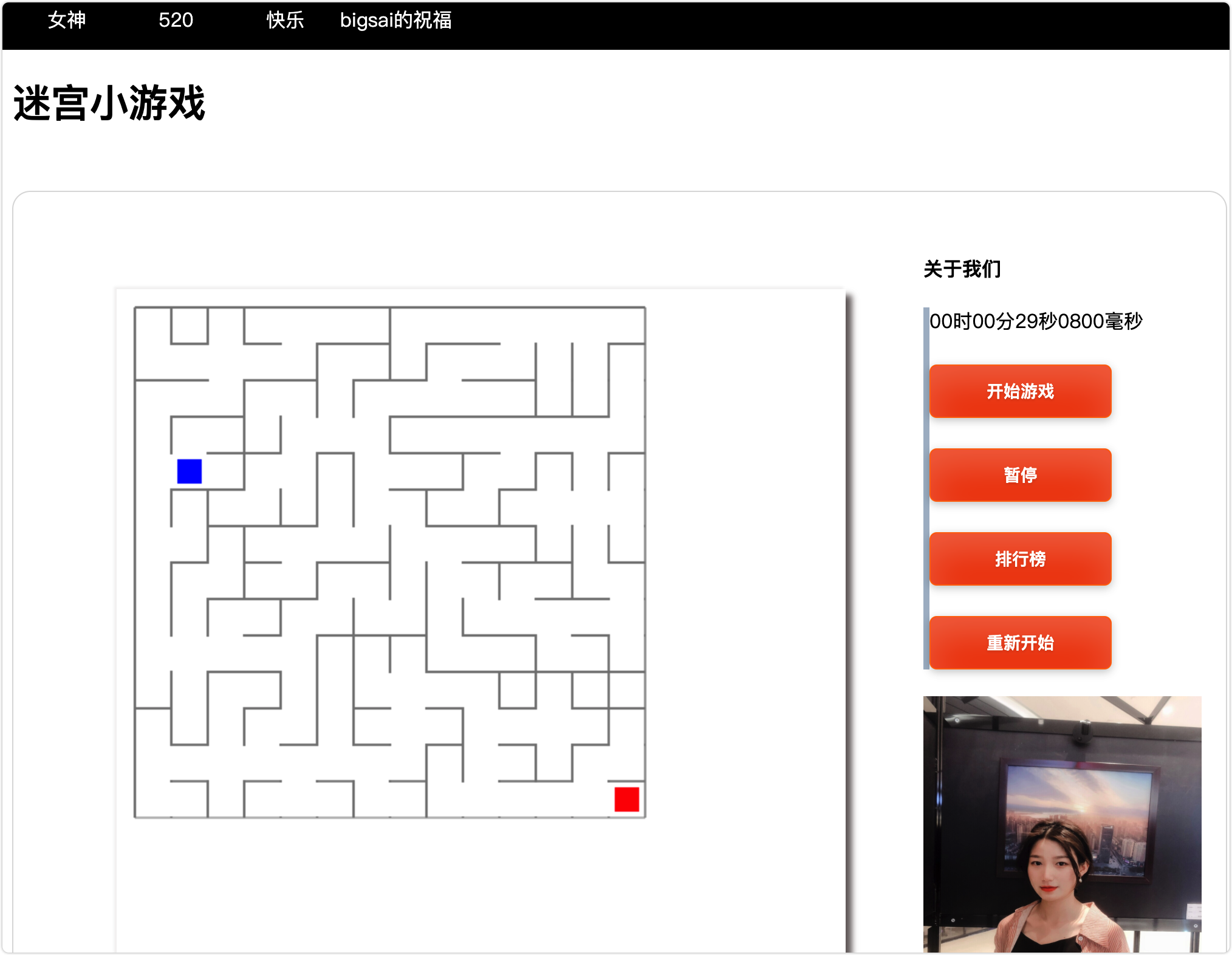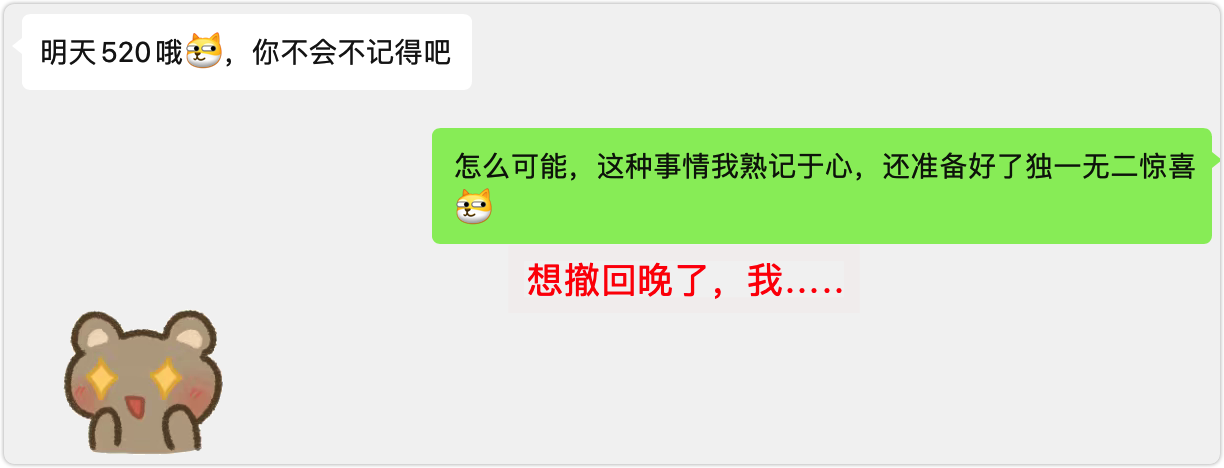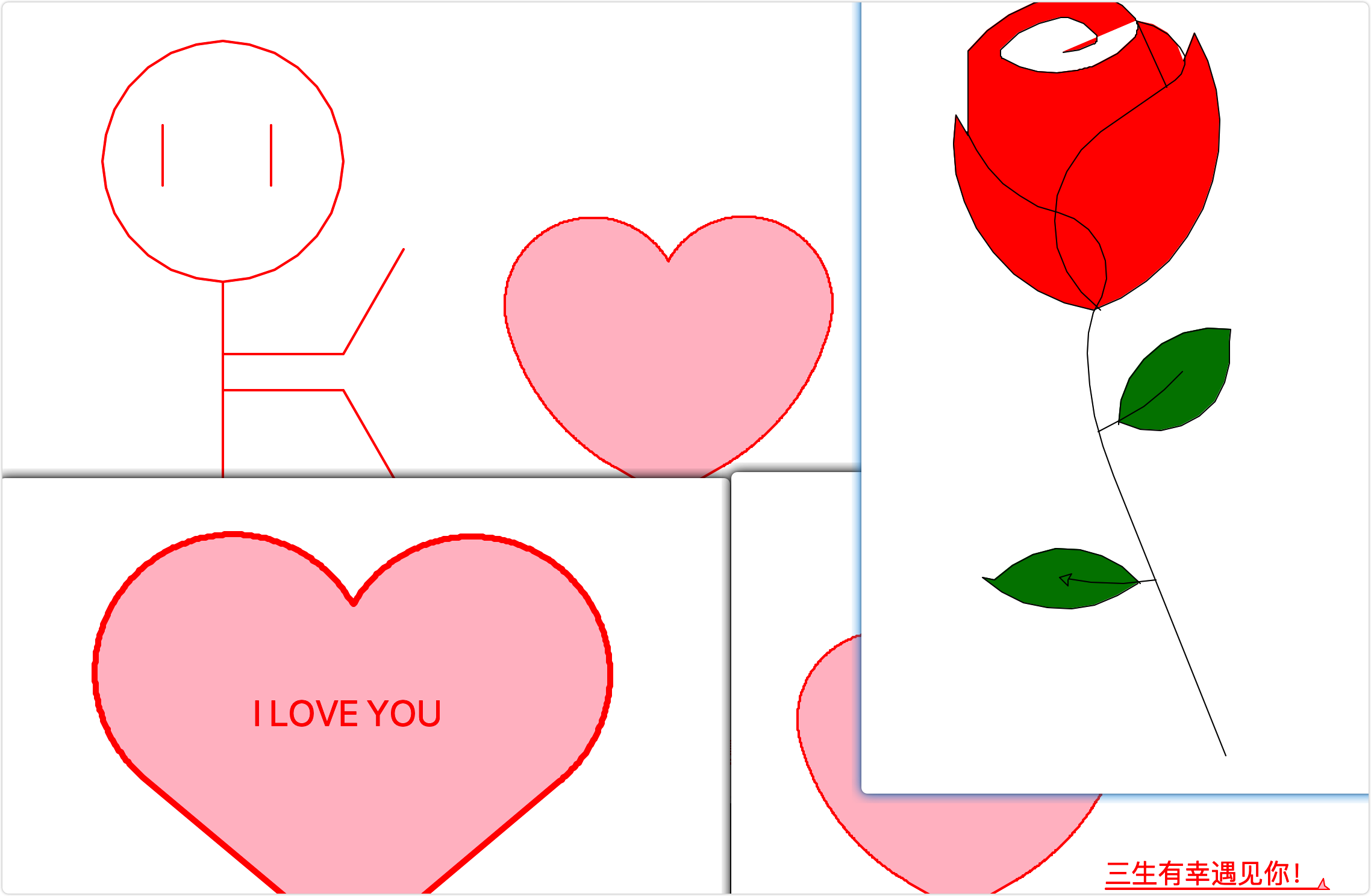### 分析

• 搞懂一個初始位置至結束位置有到達路徑的迷宮生成演算法
• 用JavaScript在canvas上畫棋盤(迷宮初始狀態)
• 利用迷宮生成演算法生成一個迷宮(擦除需要擦掉的線)
• 利用JavaScript、事件監聽和canvas畫圖實現方塊移動(要考慮不出界、不穿牆)

• 畫單條線—>畫多個線—>擦線(形成迷宮)—>方塊移動、移動約束(不出界不穿牆)—>完成遊戲

### 畫線(棋盤)

``````<!DOCTYPE html>
<html>
<title>MyHtml.html</title>
<body>
<canvas id="mycanvas" width="600px" height="600px"></canvas>
</body>
<script type="text/javascript">

var chess = document.getElementById("mycanvas");
var context = chess.getContext('2d');

function drawChessBoard(){//繪畫
context.strokeStyle='gray';//可選區域
context.moveTo(15+i*30,15);//垂直方向畫15根線，相距30px;
context.stroke();
context.moveTo(15,15+i*30);//水平方向畫15根線，相距30px;棋盤為14*14；
context.stroke();
}
}
drawChessBoard();//繪製棋盤

</script>
</html>
``````

### 畫迷宮

1：定義好不想交集合的基本類和方法（`search,union`等） 2：陣列初始化，每一個數組元素都是一個集合，值為-1 3：隨機查詢一個格子（一維資料要轉換成二維，有點麻煩），在隨機找一面牆（也就是找這個格子的上下左右），還要判斷找的格子出沒出界是否為一個合法的格子。

• 具體生成一個隨機數m(小於迷宮總格子數)
• 將一維隨機數m轉成在迷宮橫縱二維位置位置p，具體為：`[m/長,m%長]` 這裡的表示迷宮的行數或者列數。
• 在位置p的上下左右隨機找一個位置q`[m/長+1,m%長]``[m/長-1,m%長]``[m/長,m%長+1]``[m/長,m%長-1]`
• 判斷是否越界，如果越界重新查詢，否則進行下一步。

4：判斷兩個格子p和q(這時候要將二維座標轉成其一維陣列編號)是否在一個集合(並查集查詢)。如果在，則返回第三步重新找，如果不在，那麼把牆挖去。 5把牆挖去(合併)有點繁瑣，就算兩個方格不連通，需要再通過位置判斷它那種牆(上下隔離還是左右隔離)，然後再通過計算精確定位到這個牆起點末點位置然後擦掉(需要考慮相當多的細節)。 6：重複上面工作，直到第一個(1,1)和(n,n)聯通停止得到一個完整的迷宮。雖然採用隨機數找方格找牆，但是這個資料量效率和結果都還是挺不錯的。

``````while(search(0)!=search(aa*aa-1))//主要思路
{
var num = parseInt(Math.random() * aa*aa );//產生一個小於196的隨機數
var neihbour=getnei(num);
if(search(num)==search(neihbour)){continue;}
else//不在一個上
{
isling[num][neihbour]=1;isling[neihbour][num]=1;
drawline(num,neihbour);//劃線
union(num,neihbour);

}
}
``````

``````<!DOCTYPE html>
<html>
<title>MyHtml.html</title>
<body>
<canvas id="mycanvas" width="600px" height="600px"></canvas>

</body>
<script type="text/javascript">
var chess = document.getElementById("mycanvas");
var context = chess.getContext('2d');

var tree = [];//存放是否聯通
var isling=[];//判斷是否相連
tree[i]=[];
tree[i][j]=-1;//初始值為0
}
isling[i]=[];
isling[i][j]=-1;//初始值為0
}
}

function drawChessBoard(){//繪畫
context.strokeStyle='gray';//可選區域
context.moveTo(15+i*30,15);//垂直方向畫15根線，相距30px;
context.stroke();
context.moveTo(15,15+i*30);//水平方向畫15根線，相距30px;棋盤為14*14；
context.stroke();
}
}
drawChessBoard();//繪製棋盤

function getnei(a)//獲得鄰居號  random
{
var mynei=new Array();//儲存鄰居
var ran=parseInt(Math.random() * mynei.length );
return mynei[ran];

}
function search(a)//找到根節點
{
{
}
else
return a;
}
function value(a)//找到樹的大小
{
{
}
else
}
function union(a,b)//合併
{
var a1=search(a);//a根
var b1=search(b);//b根
if(a1==b1){}
else
{
{
}
else
{
}
}
}

function drawline(a,b)//劃線，要判斷是上下還是左右
{

var x3=(x1+x2)/2;
var y3=(y1+y2)/2;
if(x1-x2==1||x1-x2==-1)//左右方向的點  需要上下劃線
{
context.strokeStyle = 'white';
context.clearRect(29+x3*30, y3*30+16,2,28);
}
else
{
context.strokeStyle = 'white';
context.clearRect(x3*30+16, 29+y3*30,28,2);
}
}
{
var neihbour=getnei(num);
if(search(num)==search(neihbour)){continue;}
else//不在一個上
{
isling[num][neihbour]=1;isling[neihbour][num]=1;
drawline(num,neihbour);//劃線
union(num,neihbour);

}
}
</script>
</html>

``````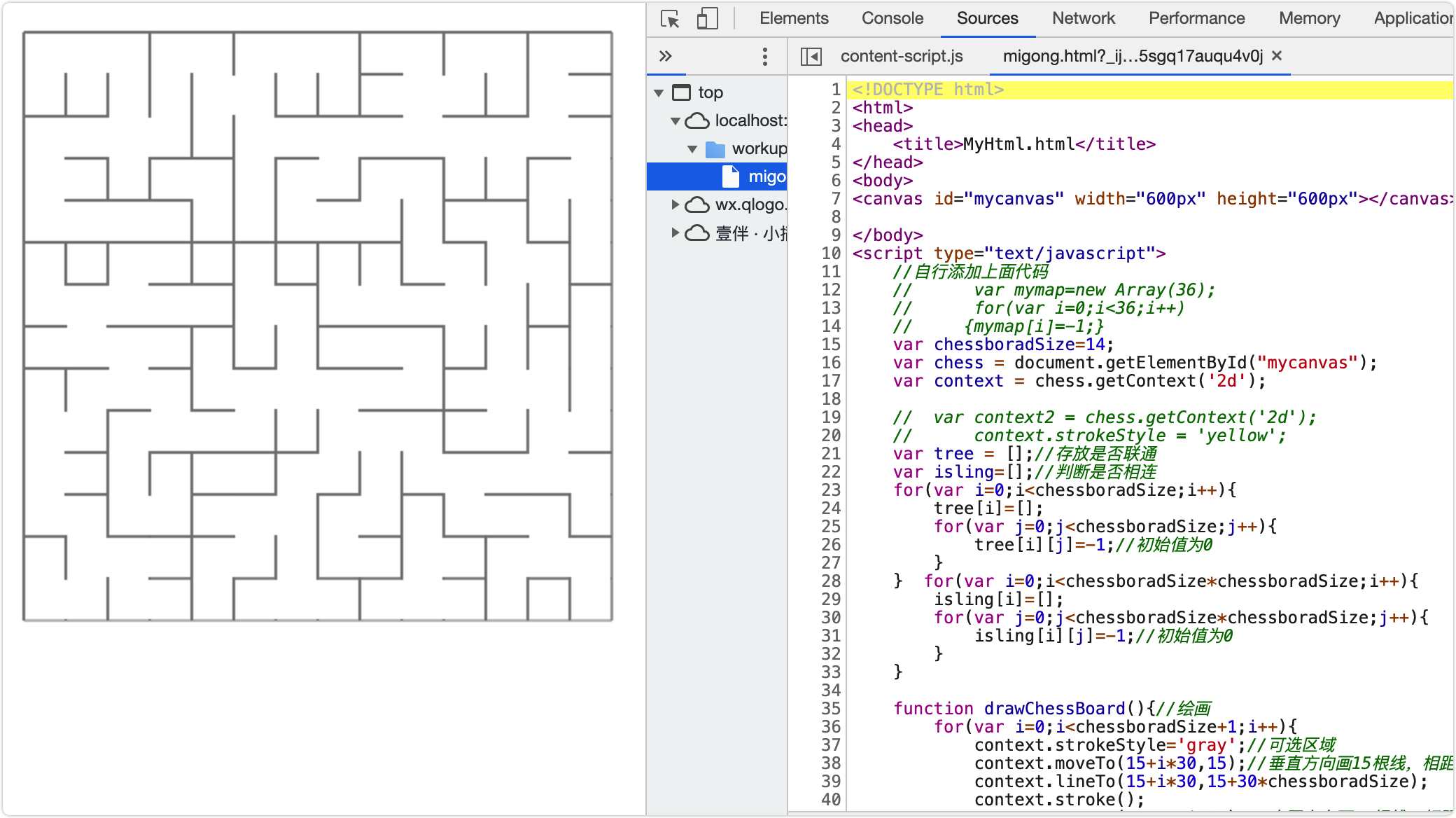### 結語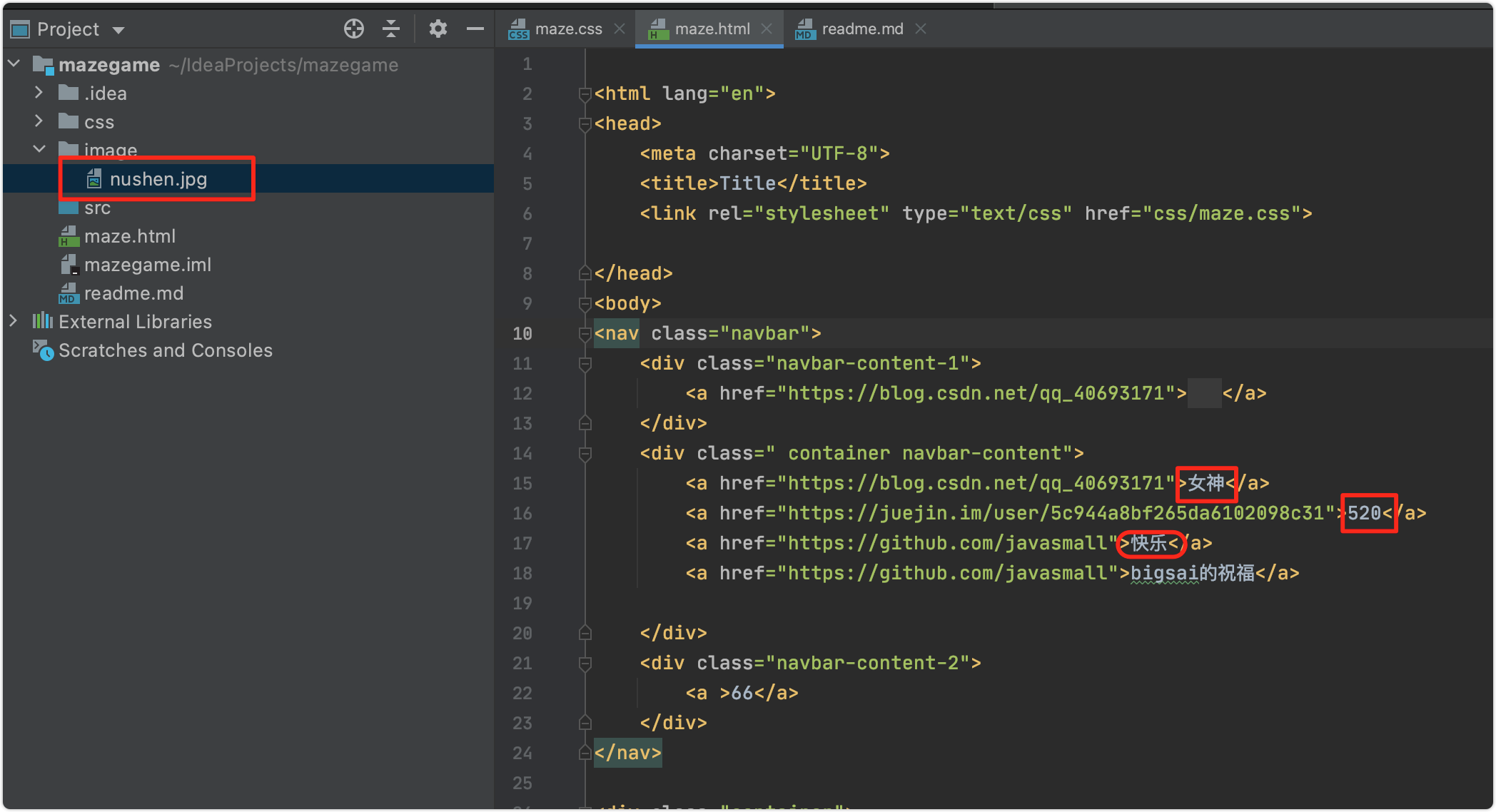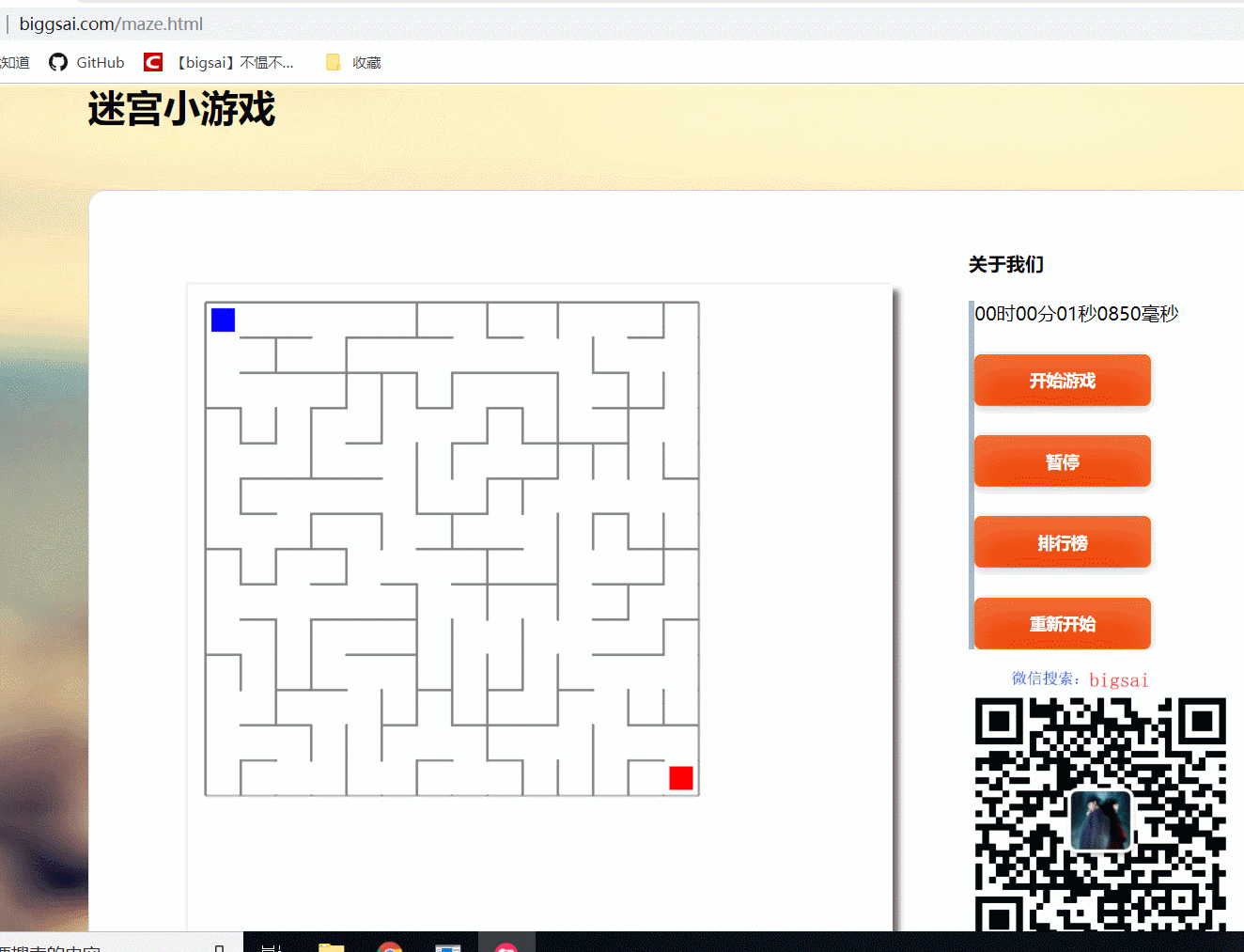「其他文章」